## Example Questions

1 2 3 4 5 6 7 8 10 Next →

### Example Question #41 : How To Solve For A Variable As Part Of A Fraction

Solve for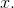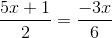Possible Answers: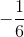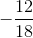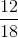Correct answer:Explanation:

To solve these types of problems, you need to cross multiply the fractions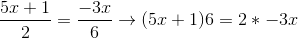.

Then, all we need to do is distribute the, combine like terms, and solve the algebraic equation.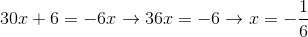.

### Example Question #42 : How To Solve For A Variable As Part Of A Fraction

Solve for: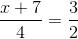Possible Answers:Correct answer:Explanation:

Cross-multiply: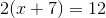Distribute: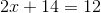Solve for x: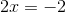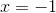### Example Question #43 : How To Solve For A Variable As Part Of A Fraction

Solve for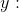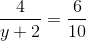Possible Answers: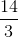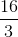Correct answer:Explanation:

Solve this question by cross-multiplying: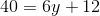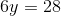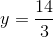### Example Question #2 : How To Solve For A Variable As Part Of A Fraction

If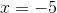, what is the value of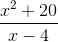?

Possible Answers: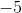Correct answer:Explanation:

To solve this question, substitute -5 in for x in the numerator and denominator. Remember that the square of a negative number is positive.

45 / -9 = -5

1 2 3 4 5 6 7 8 10 Next →

### All ACT Math Resources